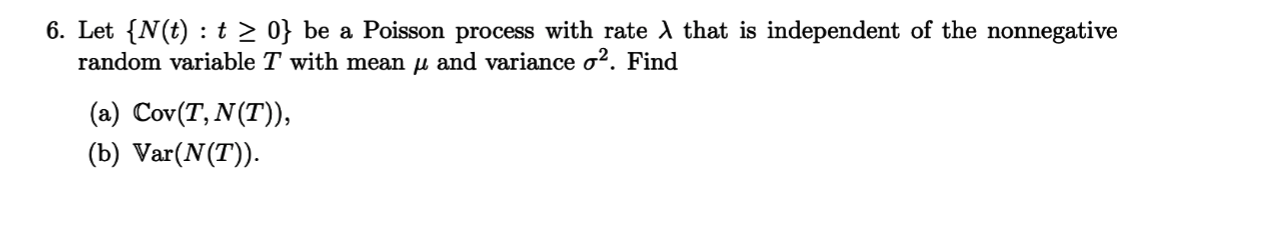# 6. Let {N(t) t 2 0} be a Poisson process with rate AX that is independent of the nonnegativerandom variable T with mean u and variance o2. Find(a) Cov(T, N(T)),(b) Var(N(T)

Question
8 viewshelp_outlineImage Transcriptionclose6. Let {N(t) t 2 0} be a Poisson process with rate AX that is independent of the nonnegative random variable T with mean u and variance o2. Find (a) Cov(T, N(T)), (b) Var(N(T) fullscreen
check_circle

Step 1

As per question, N(T) is a poisson process with rate λ and it is independent of the nonnegative random variable T with mean µ and variance σ2.

a)

T and N(T) are independent, then they are not correlated with each other, so

Cov(T,N(T))  = 0

⸪ Covariance is zero for independent variables.

Step 2

b) Variance of a poisson distribution with parameter λ is λ...

### Want to see the full answer?

See Solution

#### Want to see this answer and more?

Solutions are written by subject experts who are available 24/7. Questions are typically answered within 1 hour.*

See Solution
*Response times may vary by subject and question.
Tagged in

### Other